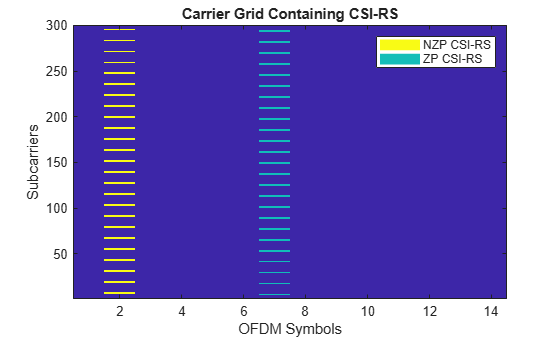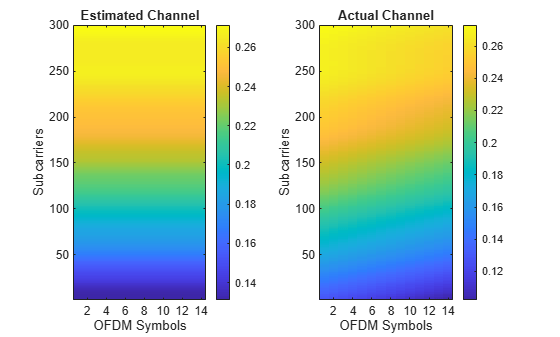# NR Channel Estimation Using CSI-RS

This example shows how to generate channel state information reference signal (CSI-RS) symbols and indices for a given carrier and CSI-RS resource configuration, as defined in TS 38.211 Section 7.4.1.5. The example shows how to map the generated symbols to the carrier resource grid, performs channel estimation at the receiver side, and compares the estimated channel against the actual channel.

### Introduction

CSI-RS is a downlink-specific (DL) reference signal. The NR standard defines zero-power (ZP) and non-zero-power (NZP) CSI-RSs.

The user equipment (UE) processes utilize NZP-CSI-RSs:

• L1-Reference signal received power (RSRP) measurements for mobility and beam management

• DL CSI acquisition

• Interference measurement

• Time and frequency tracking

ZP-CSI-RS is used for DL CSI acquisition and interference measurement. It also masks certain resource elements (REs) to make them unavailable for PDSCH transmission. As the name ZP indicates, nothing is transmitted in those REs.

This example shows how to use CSI-RS to perform channel estimation which forms the basis of CSI acquisition.

### Initialize Configuration Objects

Create a carrier configuration object representing a 5 MHz carrier with subcarrier spacing of 15 kHz.

```carrier = nrCarrierConfig; carrier.NSizeGrid = 25; carrier.SubcarrierSpacing = 15; carrier.NSlot = 1; carrier.NFrame = 0```
```carrier = nrCarrierConfig with properties: NCellID: 1 SubcarrierSpacing: 15 CyclicPrefix: 'normal' NSizeGrid: 25 NStartGrid: 0 NSlot: 1 NFrame: 0 Read-only properties: SymbolsPerSlot: 14 SlotsPerSubframe: 1 SlotsPerFrame: 10 ```

Create a CSI-RS configuration object representing two CSI-RS resources, NZP with row number 3 and ZP with row number 5.

```csirs = nrCSIRSConfig; csirs.CSIRSType = {'nzp','zp'}; csirs.CSIRSPeriod = {[5 1],[5 1]}; csirs.Density = {'one','one'}; csirs.RowNumber = [3 5]; csirs.SymbolLocations = {1,6}; csirs.SubcarrierLocations = {6,4}; csirs.NumRB = 25```
```csirs = nrCSIRSConfig with properties: CSIRSType: {'nzp' 'zp'} CSIRSPeriod: {[5 1] [5 1]} RowNumber: [3 5] Density: {'one' 'one'} SymbolLocations: { } SubcarrierLocations: { } NumRB: 25 RBOffset: 0 NID: 0 Read-only properties: NumCSIRSPorts: [2 4] CDMType: {'FD-CDM2' 'FD-CDM2'} ```

Consider the power scaling of CSI-RS in dB.

```powerCSIRS = 0; disp(['CSI-RS power scaling: ' num2str(powerCSIRS) ' dB']);```
```CSI-RS power scaling: 0 dB ```

### Generate CSI-RS Symbols and Indices

Generate CSI-RS symbols for the specified carrier and CSI-RS configuration parameters. Apply power scaling.

```sym = nrCSIRS(carrier,csirs); csirsSym = sym*db2mag(powerCSIRS);```

The variable `csirsSym` is a column vector containing CSI-RS symbols.

Generate CSI-RS indices for the specified carrier and CSI-RS configuration parameters.

`csirsInd = nrCSIRSIndices(carrier,csirs);`

The variable `csirsInd` is also a column vector of same size as that of `csirsSym`.

When you configure both ZP and NZP resources, the generation of ZP signals takes priority over the generation of NZP signals.

### Initialize Carrier Grid

Initialize the carrier resource grid for one slot.

```ports = max(csirs.NumCSIRSPorts); % Number of antenna ports txGrid = nrResourceGrid(carrier,ports);```

### Map CSI-RS Symbols Onto Carrier Grid

Perform resource element mapping.

`txGrid(csirsInd) = csirsSym;`

Plot the locations of the CSI-RS (both ZP and NZP) in the grid.

`plotGrid(size(txGrid),csirsInd,csirsSym);`### Perform OFDM Modulation

Perform OFDM modulation to generate the time-domain waveform.

`[txWaveform,ofdmInfo] = nrOFDMModulate(carrier,txGrid);`

### Pass Time-Domain Waveform Through Channel and Add AWGN Noise

Configure the number of receiving antennas.

`R = 4;`

Configure the channel.

```channel = nrTDLChannel; channel.NumTransmitAntennas = ports; channel.NumReceiveAntennas = R; channel.DelayProfile = 'TDL-C'; channel.MaximumDopplerShift = 10; channel.DelaySpread = 1e-8```
```channel = nrTDLChannel with properties: DelayProfile: 'TDL-C' DelaySpread: 1.0000e-08 MaximumDopplerShift: 10 SampleRate: 30720000 MIMOCorrelation: 'Low' Polarization: 'Co-Polar' TransmissionDirection: 'Downlink' NumTransmitAntennas: 4 NumReceiveAntennas: 4 NormalizePathGains: true InitialTime: 0 NumSinusoids: 48 RandomStream: 'mt19937ar with seed' Seed: 73 NormalizeChannelOutputs: true ```

Based on the configured channel, append zeros to the transmitted waveform to account for the channel delay.

```chInfo = info(channel); maxChDelay = ceil(max(chInfo.PathDelays*channel.SampleRate)) + chInfo.ChannelFilterDelay; txWaveform = [txWaveform; zeros(maxChDelay,size(txWaveform,2))];```

Pass waveform through channel.

`[rxWaveform,pathGains] = channel(txWaveform);`

To produce the actual propagation channel `H_actual`, perform perfect channel estimation.

```pathFilters = getPathFilters(channel); H_actual = nrPerfectChannelEstimate(carrier,pathGains,pathFilters);```

Add AWGN noise to the waveform.

```SNRdB = 50; % in dB SNR = 10^(SNRdB/20); % Linear value N0 = 1/(sqrt(2.0*R*double(ofdmInfo.Nfft))*SNR); % Noise variance rng(0); noise = N0*complex(randn(size(rxWaveform)),randn(size(rxWaveform))); rxWaveform = rxWaveform + noise```
```rxWaveform = 7690×4 complex 0.0000 - 0.0001i -0.0001 + 0.0000i -0.0001 - 0.0000i -0.0001 + 0.0000i 0.0001 - 0.0001i -0.0000 + 0.0000i 0.0001 - 0.0000i -0.0000 - 0.0000i -0.0001 + 0.0000i 0.0001 + 0.0000i -0.0001 - 0.0000i -0.0001 + 0.0000i 0.0000 - 0.0000i 0.0000 - 0.0000i -0.0001 + 0.0001i -0.0000 - 0.0000i 0.0000 + 0.0001i 0.0001 - 0.0000i 0.0000 - 0.0000i 0.0001 - 0.0000i -0.0001 + 0.0000i -0.0000 + 0.0000i -0.0000 + 0.0000i 0.0001 + 0.0000i -0.0000 - 0.0001i -0.0000 - 0.0001i -0.0001 - 0.0000i -0.0000 - 0.0000i 0.0000 + 0.0001i -0.0001 - 0.0000i -0.0000 + 0.0000i -0.0000 - 0.0000i 0.0002 - 0.0001i 0.0001 + 0.0001i 0.0001 + 0.0000i 0.0001 + 0.0000i 0.0001 + 0.0000i 0.0000 + 0.0000i 0.0000 - 0.0001i 0.0000 - 0.0001i ⋮ ```

Perform timing synchronization using NZP-CSI-RS. To estimate timing offset, use `nrTimingEstimate` and consider the NZP-CSI-RS as a reference.

```% Disable ZP-CSI-RS resource, not going to be used for timing and channel % estimation csirs.CSIRSPeriod = {[5 1],'off'}; % Generate reference symbols and apply power scaling refSym = db2mag(powerCSIRS)*nrCSIRS(carrier,csirs); % Generate reference indices refInd = nrCSIRSIndices(carrier,csirs); offset = nrTimingEstimate(carrier,rxWaveform,refInd,refSym)```
```offset = 7 ```
`rxWaveform = rxWaveform(1+offset:end,:);`

OFDM demodulate the received time-domain waveform.

`rxGrid = nrOFDMDemodulate(carrier,rxWaveform); % Of size K-by-L-by-R`

### Compare Estimated Channel Against Actual Channel

Perform practical channel estimation using NZP-CSI-RS. Make sure the CSI-RS symbols `csirsSym` are of the same CDM type.

```cdmLen = [2 1]; % Corresponds to CDMType = 'FD-CDM2' [H_est,nVar] = nrChannelEstimate(carrier,rxGrid,refInd,refSym,'CDMLengths',cdmLen); estSNR = -10*log10(nVar); disp(['estimated SNR = ' num2str(estSNR) ' dB'])```
```estimated SNR = 27.4577 dB ```

Plot the estimated channel and actual channel between first transmitting antenna and first receiving antenna.

```figure; % Plot the estimated channel subplot(1,2,1) imagesc(abs(H_est(:,:,1,1))); colorbar; title('Estimated Channel') axis xy; xlabel('OFDM Symbols'); ylabel('Subcarriers'); % Plot the actual channel subplot(1,2,2) imagesc(abs(H_actual(:,:,1,1))); colorbar; title('Actual Channel') axis xy; xlabel('OFDM Symbols'); ylabel('Subcarriers');```Calculate the channel estimation error.

```H_err = (H_est - H_actual(:,:,:,1:size(H_est,4))); [minErr,maxErr] = bounds(abs(H_err),'all'); disp(['Absolute value of the channel estimation error is in the range of [' num2str(minErr) ', ' num2str(maxErr) ']'])```
```Absolute value of the channel estimation error is in the range of [4.9184e-06, 0.035047] ```

### Local Functions

```function plotGrid(gridSize,csirsInd,csirsSym) % plotGrid(GRIDSIZE,CSIRSIND,CSIRSSYM) plots the carrier grid of size GRIDSIZE % by populating the grid with CSI-RS symbols using CSIRSIND and CSIRSSYM. figure() cmap = colormap(gcf); chpval = {20,2}; chpscale = 0.25*length(cmap); % Scaling factor tempSym = csirsSym; tempSym(tempSym ~= 0) = chpval{1}; % Replacing non-zero-power symbols tempSym(tempSym == 0) = chpval{2}; % Replacing zero-power symbols tempGrid = complex(zeros(gridSize)); tempGrid(csirsInd) = tempSym; image(chpscale*tempGrid(:,:,1)); % Multiplied with scaling factor for better visualization axis xy; names = {'NZP CSI-RS','ZP CSI-RS'}; clevels = chpscale*[chpval{:}]; N = length(clevels); L = line(ones(N),ones(N),'LineWidth',8); % Generate lines % Index the color map and associate the selected colors with the lines set(L,{'color'},mat2cell(cmap( min(1+clevels,length(cmap) ),:),ones(1,N),3)); % Set the colors according to cmap % Create legend legend(names{:}); title('Carrier Grid Containing CSI-RS') xlabel('OFDM Symbols'); ylabel('Subcarriers'); end```

 3GPP TS 38.211. “NR; Physical channels and modulation.” 3rd Generation Partnership Project; Technical Specification Group Radio Access Network.